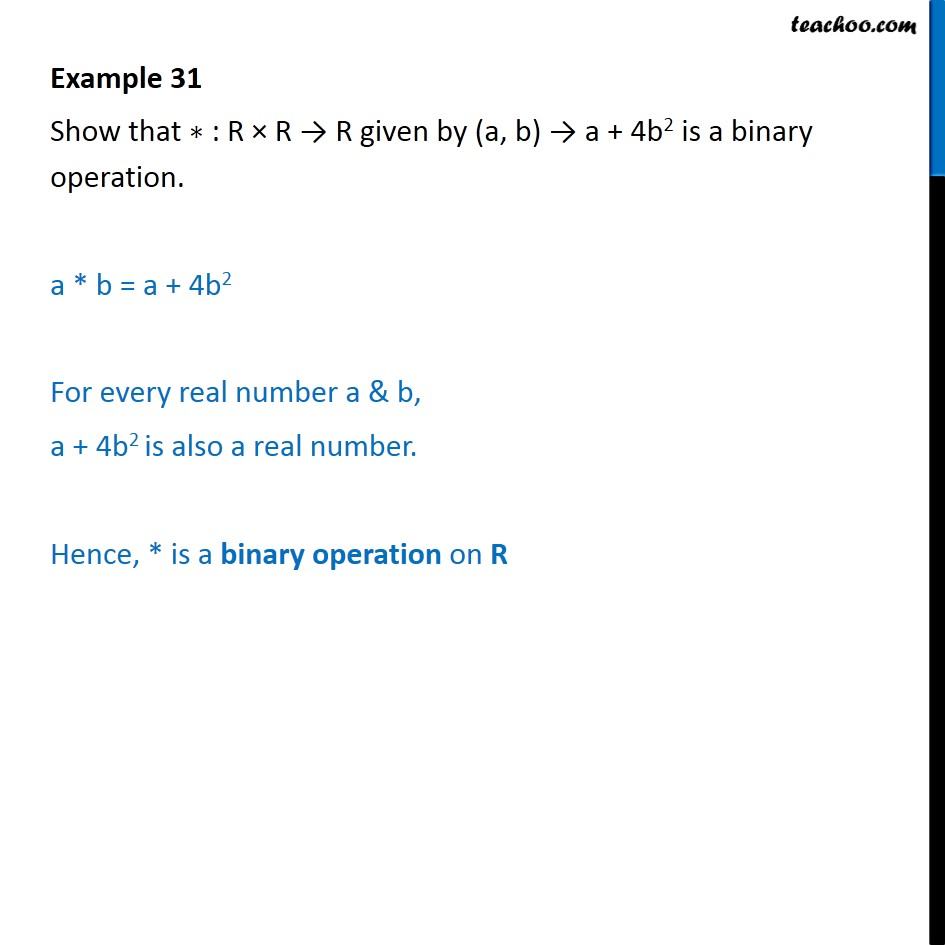1. Chapter 1 Class 12 Relation and Functions
2. Serial order wise
3. Examples

Transcript

Example 31 Show that : R R R given by (a, b) a + 4b2 is a binary operation. a * b = a + 4b2 For every real number a & b, a + 4b2 is also a real number. Hence, * is a binary operation on R

Examples